# Fault Analysis of Three Level VSC-HVDC Connected Offshore Wind

Fault Analysis of Three Level VSC-HVDC Connected Offshore Wind
Laboratoire de Maitrise des Energies Renouvelables (LMER), Faculté de Technologie, Université de Bejaia, Bejaia 06000, Algeria

Corresponding Author Email:
Page:
425-432
|
DOI:
https://doi.org/10.18280/mmep.100206
7 November 2022
|
Revised:
26 January 2023
|
Accepted:
3 February 2023
|
Available online:
28 April 2023
| Citation

OPEN ACCESS

Abstract:

High-voltage direct current (HVDC) system is widely used for long-distance bulk-power transmission due to its high economic efficiency. VSC-HVDC systems are being increasingly employed in the power systems. This paper addresses the analysis, fault of three level VSC HVDC connected offshore wind. Where wind energy can be very effectively used and is currently widely used worldwide. The onshore VSC-HVDC system is based on a three-level voltage converter topology that is used to interconnect an offshore wind farm (OWF) with the ac main grid via two dc cables. The VSC consists three-phase insulated-gate bipolar transistor (IGBT) based rectifier, a three-phase IGBT based inverter. The modeling of dc bus voltage of inverter is developed via the Voltage Oriented Control (VOC) strategy. AC fault three-lines to ground and line to ground, DC fault with pole-to-ground type on DC transmission lines are mainly considered and analyzed. The system is simulated using Matlab/Simulink environment; the simulation results show the control efficiency and system stability. The results obtained confirm the robustness of the system control and the system gives a good energy quality that manifests by a good output currant and voltage curves.

Keywords:

offshore wind farm, VSC, high voltage direct current, AC and DC faults, stability, power quality

1. Introduction

Global energy consumption continues to grow with no prospect of slowing down in the near future . Continued growth in demand for electricity requires the continued expansion of plans to increase production capacity, and that of transport [2, 3], and to promote the interconnection of regions, which are sometimes separated by long distances. There is a need to transmit energy through the sea [4, 5], and the interconnection of asynchronous systems of different frequencies. HVDC transport, therefore, emerges as the only possible solution responding to the various problems and drawbacks encountered with the alternating current for these environmental [6, 7], technical, and economic reasons. The installation of HVDC systems is favored in order to maximize the efficiency of electricity transmission [8, 9]. Technically, the interconnection of these wind farms with electric grids provides a better quality of power, an improvement in the transition of power, and stability of the network [10, 11]. Recent advancements in VSC technology have made HVDC grids more technically feasible.

The HVDC transmission method makes use of technology that was substantially developed in the 1930s in Germany and Sweden (ASEA). Early commercial installations included one between Moscow and Kashira in the Soviet Union in 1951 and one between Gotland and the Swedish mainland in 1954.The previous longest HVDC link in the world was the Xiangjiaba–Shanghai 2,071 km (1,287 mi), ±800 kV, 6400 MW link connecting the Xiangjiaba Dam to Shanghai. in the People's Republic of China. Early in 2013, the longest HVDC link has been the Rio Madeira link in Brazil, which consists of two bipoles of ±600 kV, 3150 MW each, connecting Porto Velho in the state of Rondônia to the Sao Paulo area, over a length of 2,375 km. Rock Island Clean Line was being installed in North America, over a length of 805 km, and power of 3,500 MW which is expected to be completed in the year 2017. It requires additional research and development on various faults and components to operate at the extreme .

However, under a fault in the DC or AC side, an over current may occur due to the rapid decrease in the DC voltage. This is mainly because the conventional VSCs cannot interrupt or strongly limit the current flowing towards the DC-side fault. Due to the freewheeling diodes feeding the DC fault, a strong AC grid current flows through them, potentially damaging the converter valves. Recently, protecting HVDC transmission lines using VSC has proven to be very difficult [13-15].

The major priority of an HVDC is maximum power transfer and system stability. when a fault appears, stability is affected and a loss of power is visible. So the study and analysis of the different faults allows us to understand the reaction of the HVDC system to faults and to adapt protection systems or controls.

DC and AC fault analysis are always interesting to understand the system behavior under such conditions. Furthermore, the analysis of the different faults in an HVDC system allows better protection and understanding with a lower cost.

Fault analysis is an important part of any system's defense. HVDC systems must be operated safely, the detection and quick correction of faults in HVDC lines are critical [16, 17]. AC side failures on the rectifier and inverter sides, as well as DC faults on the line, can occur in HVDC systems. For improved system protection, it is required to evaluate and analyze the fault . However, all the studies that have been carried out so far are faults on the AC side or faults on the DC side [DC phase-ground, DC phase- phase or AC faults.

The focus of this work is the examination of several sorts of short circuits and stability in offshore wind farm (WF) of a HVDC system. The analysis will consider three‐levels voltage source converter (VSC). VSC HVDC is normally designed to ride through all AC faults.

This work is part of the perspective of studying the performance and stability of a VSC-HVDC system based on a three-level inverter. Thus, simulations of the studied VSC-HVDC system were carried out in Simpower System of Matlab, the control of this system is based on the vector oriented control.

This article is organized in four sections: First, a description of the system with a mathematical model is made. Section 2, we present and detail the control system. Then section 3, simulations of the system are carried out under different faults in the AC and DC network. Finally, section 4, the results obtained and the performances of the VSC-HVDC system are analyzed and discussed.

2. Wind Farm (WF) and (VSC-HVDC) System Description

Figure 1 depicts the configuration of the studied system, containing a wind farm as a power source and an infinite network, connected to a transmission link of 75 km, via two smoothing capacitors and three levels VSC neutral point clamped converter (NPC) converters.

## 1.png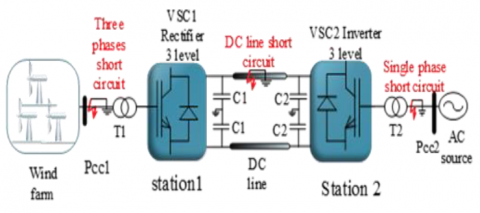Figure 1. Different faults of the system studied (VSC-HVDC)

The mathematical expression model of the wind turbine was found in research .

${{P}_{m}}=\frac{1}{2}\rho A{{C}_{p}}\left( \lambda ,\beta \right)V_{Wind}^{3}$     (1)

$\lambda =\frac{RC}{{{V}_{Wind}}}$      (2)

${{C}_{p}}=0.73\left( \frac{151}{A}0.5\beta 0.02{{\beta }^{2.14}}13.2 \right){{e}^{\frac{18.4}{A}}}$     (3)

$A=\frac{1}{\frac{1}{\lambda-0.02 \beta} \,\frac{0.003}{\beta^3-1}}$      (4)

where, ρ is the density of the air (kg/m2); A is the rotor swept area; Cp is the efficiency of the turbine; λ is the turbine tip speed ratio; β is the pitch angle of the turbine; R is the blade length; Vwind is the actual speed of the wind; is the speed of rotation of dq reference frame.

3. Control Strategy

The Figure 2 shows our system; it consists of a wind farm, DC link and two stations (St1, St2) of conversion (rectifier, inverter). We have used, the 3L-NPC based IGBT for both VSC stations to reduce converter stress and improve power quality by supporting high voltage.

## 2.png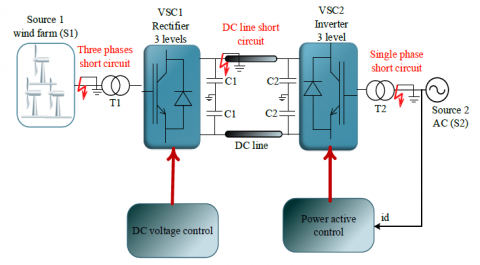Figure 2. Control structure of the three levels VSC-HVDC system

In order to maintain the power constant, the losses in the rectifier are zero and for steady state operation, the active power on the network side is the same as the active power on the DC bus. As illustrated in Figure 4, the measured DC link voltage is compared to the reference, and the error is input into a PI controller to obtain the current in the d-axis.

The following are the equations that characterize the onshore VSC-HVDC Station in the dq reference frame as shown in the research :

${{P}_{cc}}={{V}_{d}}{{i}_{d}}+{{V}_{q}}{{i}_{q}}$      (5)

${{Q}_{cc}}={{V}_{d}}{{i}_{q}}-{{V}_{q}}{{i}_{d}}$      (6)

${{V}_{d}}=L\frac{d{{i}_{d}}}{dt}+R{{i}_{d}}-WL{{i}_{q}}+{{e}_{d}}conv$      (7)

${{V}_{q}}=L\frac{d{{i}_{q}}}{dt}+R{{i}_{d}}+WL{{i}_{d}}+{{e}_{q}}conv$     (8)

${{P}_{dc}}={{V}_{dc}}{{i}_{dc}}+{{C}_{dc}}\frac{d{{V}_{dc}}}{dt}{{V}_{dc}}$      (9)

$\frac{{{V}_{dc}}}{dt}=\frac{d{{V}_{DC}}}{dt}=\frac{d{{n}_{d}}}{C}{{i}_{d}}+\frac{d{{n}_{q}}}{C}{{i}_{q}}$       (10)

where, Pa, Qcc, idq, Vdq, L, R, Cdc, Vdc, w represent the active power, the reactive power, the current, the voltage, the inductance, the resistor of the line of the onshore VSC.

${{P}_{cc}}={{V}_{d}}{{i}_{d}}$     (11)

${{Q}_{cc}}=-{{V}_{q}}{{i}_{d}}$      (12)

$i_{d}^{*}=\left( V_{dc}^{*}-{{V}_{dc}} \right)KP{{V}_{dc}}+\frac{Ki{{V}_{dc}}}{S}$     (13)

id is the reference current.

1/S Integrator, V*dc is the estimated dc-bus voltage at do not begin a new section directly at the bottom of the page, instead, move the heading to the top of the next page.

Offshore VSC Station 1, KPVdc, KiVdc is the integral gain for the regulated dc bus voltage. A detailed model of three levels is used and its parameters are reported in Table 1.

Table 1. VSC-HVDC system parameters

 Symbol Quantity Conversion in SI a system Vac Rated (base) AC voltage 150 e3 V Vdc DC voltage 300 e3 V P Active power 1000 e6 W Cdc DC capacitor 3000 e-6 f System frequency 60 Hz
4. Simulation and Discussion

The system presented in Figure 1 was implemented and simulated using Matlab/Simulink environment Simulation results are presented and discussed in what follow.

4.1 Three lines to ground (3L-G) short circuit

At the WF connection point, a 3L-G short circuit ground fault was applied for 200ms. Figures 1 and 2 show the simulation findings (3-9).

In this test, three-phase to ground fault was applied in Station 1. A change in the d axis current is introduced in St2 between 1.1 and 1.3 second in the steady state. After that the fault was applied at t=2s and lasts for 0.1s.

Figures 3 and 4 show the active and reactive powers of two stations we can see any change in the St2.

## 3.png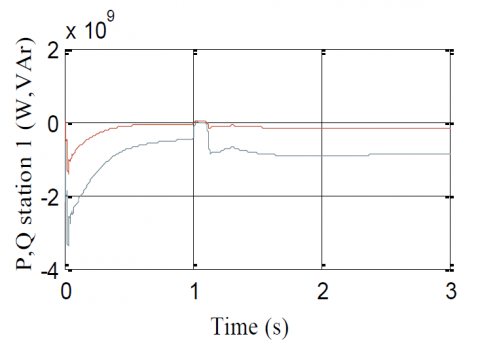Figure 3. Active and reactive power in Station 1

## 4.png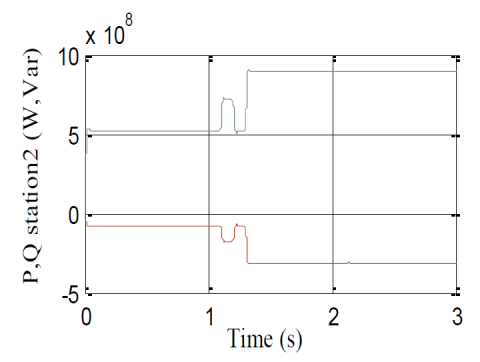Figure 4. Active and reactive power in Station 2

## 5.png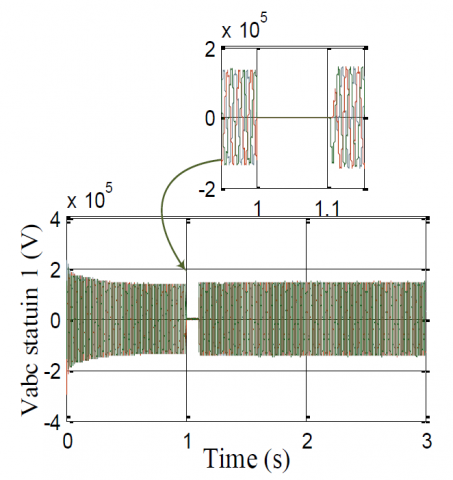Figure 5. AC-side voltage in Station 1

## 6.png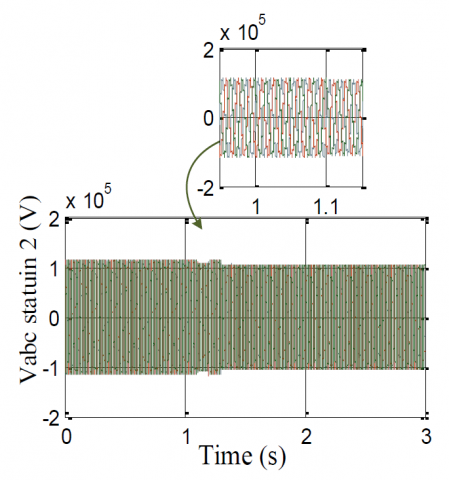Figure 6. AC-side voltage Station 2

## 7.pngFigure 7. AC -side current in Station 1

## 8.png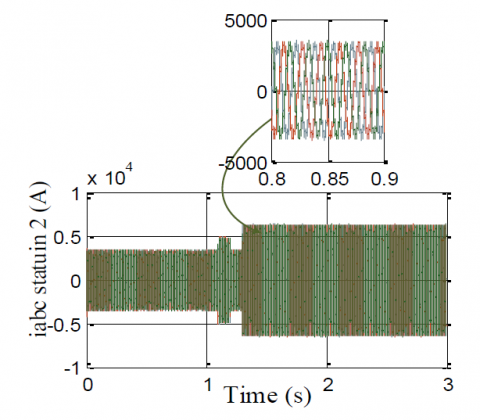Figure 8. AC- side current in Station 2

## 9.png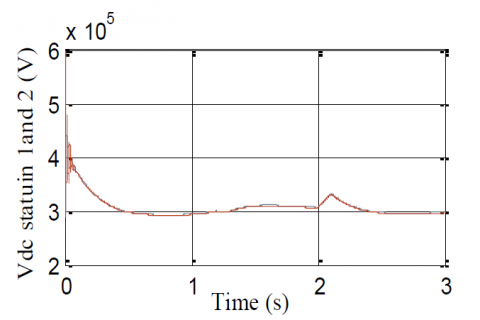Figure 9. DC voltage in Station 1 and Station 2

The line voltages and current of two stations are show in Figures 5, 6, 7 and 8 as can be seen in Figure 5 the line voltages of St1 became null because of the short circuit but the voltage in St2 is not affected. On the other hand, the short circuit causes excessive line current in St1 without any perturbation in St2 electrical quantities. These results confirm the good performances of the control algorithm. the current of the 1 station is affected by the fault, however the other station has not been affected. The DC bus test is made unstable in this short period but it stabilizes after the fault is cleared. The two voltages Vdc1 and Vdc2 (Figure 9) are completely equal, which highlights the performance and efficiency of the regulation.

4.2 Line to ground (L-G)

We applied a phase-to-earth fault at time t=2s at t=2.1s at Station 2, we find the following results.

## 10.png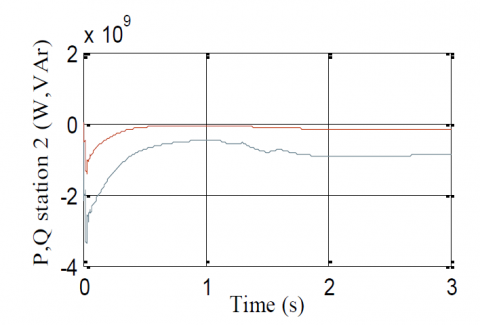Figure 10. Active and reactive power in Station 1

## 11.png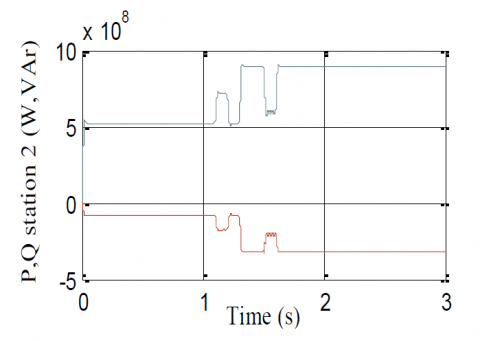Figure 11. Active and reactive power in Station 2

## 12.png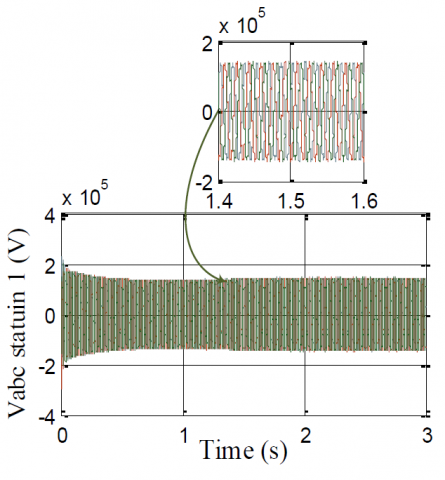Figure 12. AC-side voltage in Station 1

## 13.png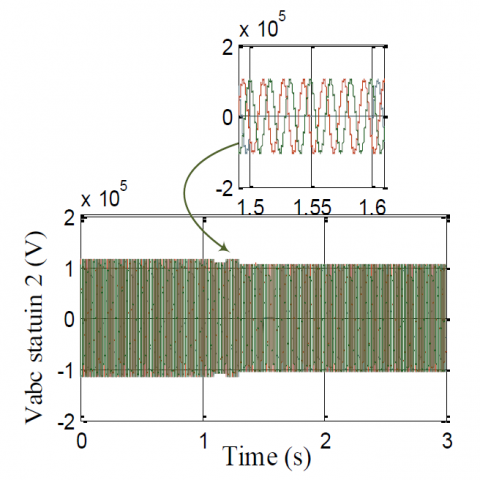Figure 13. AC- side voltage in Station 2

## 14.pngFigure 14. AC-side current in Station 1

## 15.png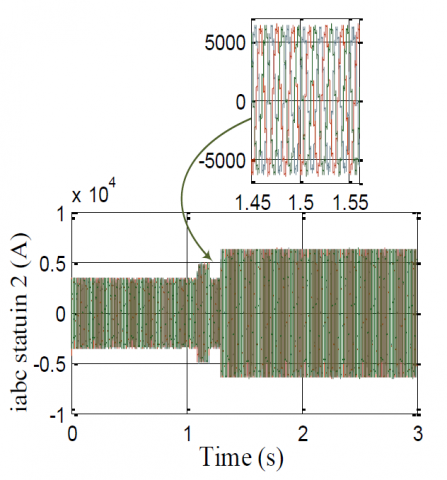Figure 15. AC-side current in Station 2

## 16.png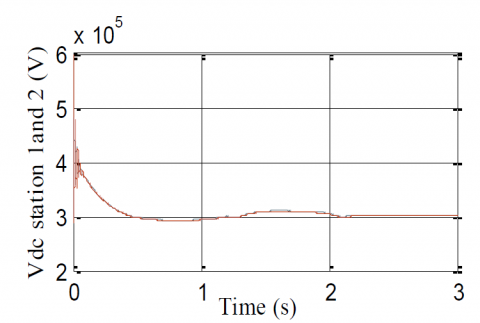Figure 16. V Dc link in Station 1 and Station 2

The fault time starts from t=2 to t=2.1 second, Figure 10 shows the variation of power in two stations, we can see the disturbance in St2 while St1 has not been affected Figure 11. From the period 2 to 2.1 second, the failure affected phase A. The three-phase voltage has a usual value of 150 kV. Figures 12 and 13 shows the AC voltage in the two stations, in the second station we can clearly see that phase A goes to 0 for 2 periods 2 s at 2.1 while the other two phases remain unchanged, On the other hand, the voltage in St1 has not been affected.

Figures 14, 15 show the current in the two stations, the current in St2 increases slightly and decreases when the fault occurs at t = 2s and ends at t = 2.1s. The current in St1 remains stable.

The DC bus test is made unstable in this short period of time but it stabilizes after the fault is cleared in Figure 16.

4.3 Line to ground (L-G)

We applied a DC line to ground fault at time t=2s at t=2.1s at St2, we find the following results.

Figures 17-23 illustrate the impacts of the pole to earth fault on the measured AC voltage and current signals at Stations 1 and 2.

## 17.png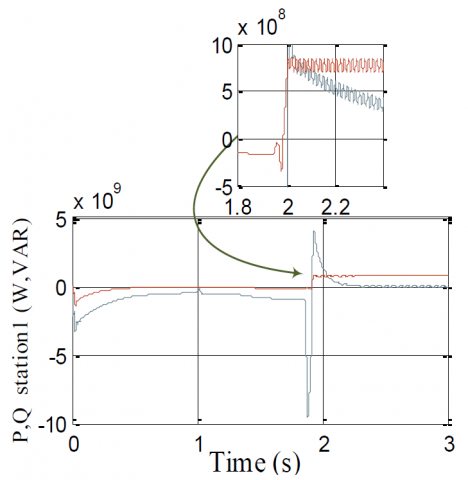Figure 17. Active and reactive power in Station 1

## 18.png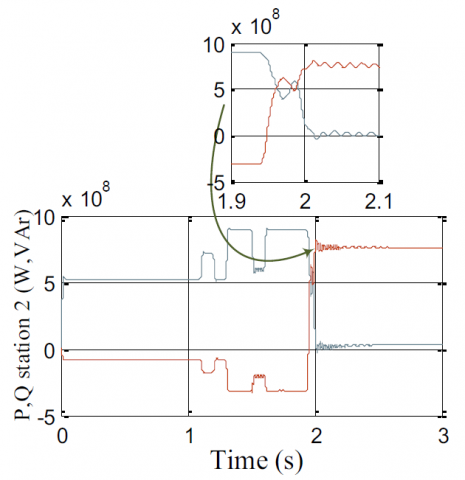Figure 18. Active and reactive power in Station 2

## 19.png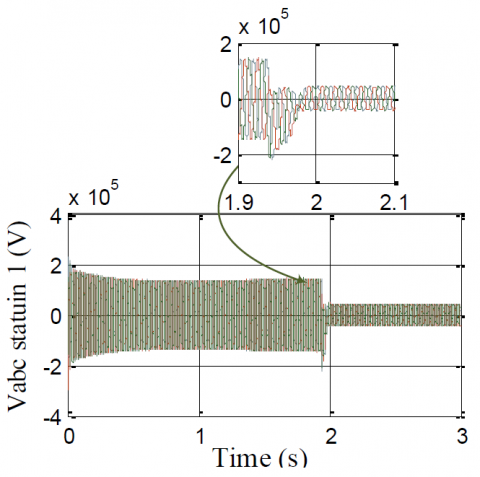Figure 19. AC-side voltage in Station 1

## 20.png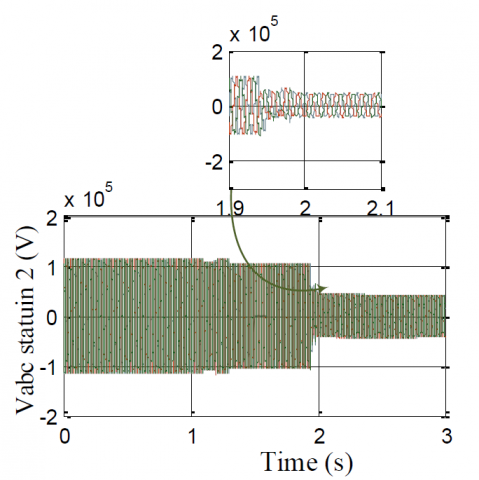Figure 20. AC-side voltage Station 2

## 21.png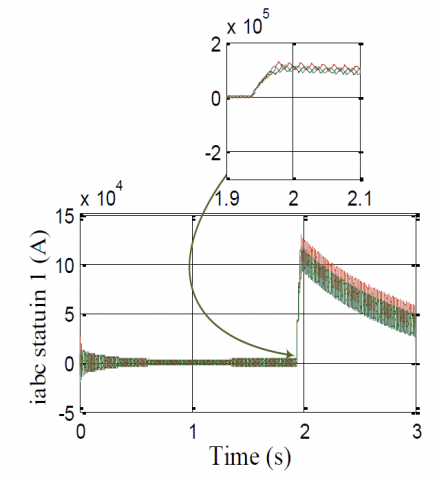Figure 21. AC-side current in Station 1

When the fault is produced on the continuous line next to Station 2 at t=2s the alternating voltage measured at the transformer presents significant distortions as shown in Figures 19 and 20 respectively.

As shown from the voltage signals corresponding to Pcc1 have greater DC offset and distortions compared to the voltage signals recorded before T1 since the AC filter installed after "T1" rejects most of the distortions. As shown from the corresponding voltage signals before T have greater DC offset and distortions compared to the voltage signals recorded after T1 since the AC filter installed after "T1" rejects most of the distortions. The DC voltage collapsed after the fault occurred as shown in Figure 23. On the other hand, the measured AC signals at station “S2" are illustrated in Figures 17-22 Distorted voltages. More distorted currents after the fault occurrence were recorded as shown in Figures 21-22, the DC current increased rapidly after the fault instant and the DC voltage collapsed remarkably.

## 22.png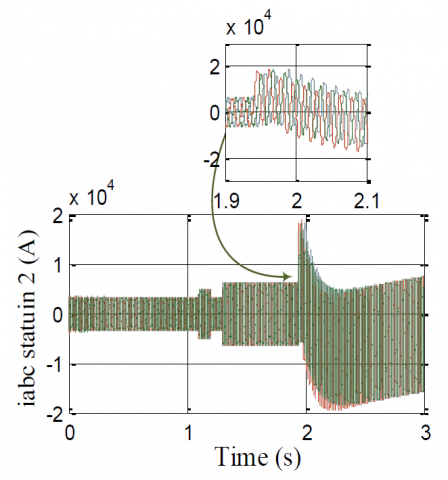Figure 22. AC-side current in Station 2

## 23.png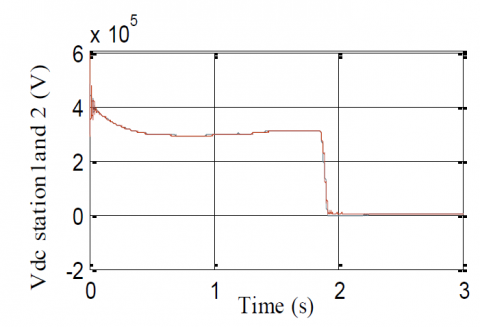Figure 23. DC voltage link in Station 1 and Station 2

5. Conclusions

This work presents, describes, and simulates the VSC-HVDC system based on three levels inverter and the vector control strategy of active power, DC bus voltage, under various situations (steps on input powers, AC side voltage, etc.) and disturbances (three-phase/ground short-circuit fault). We used the 3-level inverter since it offers less current ripple, a reduced THD value and it allows power segmentation. The findings demonstrate that the system control has good performance in terms of stability and robustness during a three-phase and single-phase short-circuit fault on the AC side. Alternating currents are observed to grow on the failing side while decreasing on the healthy side, not causing a change in the DC bus voltage. DC side faults are also presented in this article. When a fault occurs on the DC side, its impacts appear on the AC side. During a DC fault, the fault DC current increases sharply and will reach the blocking threshold value in a few milliseconds. Therefore, it is considered one of the most dangerous faults in any transmission system. With this, we can direct future research towards an HVDC transmission line protection scheme that can be implemented more reliably, efficiently and robustly and also prevent losses, predict future faults, easily control and eradicate them. Suitable appropriate protection schemes and circuit breakers can be a very good solution.

Acknowledgment

We would like to thank the Directorate general for scientific research and technological development for funding our Laboratory.

Nomenclature
 AC Alternative Current T Power transformer Cdc Dc Capacitor DC Direct Current f System frequency HVDC High Voltage Direct Current Pcc Point of common coupling VSC Voltage Source Inverter Ki Integral Kp Proportional VOC Vector Oriented Control Cp Efficiency of the turbine NPC Neutral Point Clamped Converter OWF Offshore Wind Farm IGBT Iinsulated-Gate Bipolar Transistor Greek symbols $\rho$ Density of the air $\beta$ the pitch angle λ Turbine tip speed ratio Ω Speed of rotation of dq reference frame Subscripts ac Alternative current index q Quadratic axis d Direct Axis dc Direct current index T Transformer
References

 Benadja, M., Rezkallah, M., Benhalima, S., Hamadi, A., Chandra, A. (2019). Hardware testing of sliding mode controller for improved performance of VSC-HVDC based offshore wind farm under DC fault. IEEE Transactions on Industry Applications, 55(2): 2053-2063. https://doi.org/10.1109/TIA.2018.2878539

 Jovcic, D. (2008). Offshore wind farm with a series multiterminal CSI HVDC. Electric Power Systems Research, 78(4): 747-755. https://doi.org/10.1016/j.epsr.2007.05.023

 Yahiaoui, A., Iffouzar, K., Ghedamsi, K., Himour, K. (2021). Dynamic performance analysis of VSC-HVDC based modular multilevel converter under fault. Journal Européen des Systèmes Automatisés, 54(1): 187-194. https://doi.org/10.18280/jesa.540121

 Marvik, J.I., Svendsen, H.G. (2013). Analysis of grid faults in offshore wind farm with HVDC connection. Energy Procedia, 35: 81-90. https://doi.org/10.1016/j.egypro.2013.07.161

 Hassanpoor, A., Häfner, Y., Nami, A., Vinothkumar, K. (2016). Cost-effective solutions for handling dc faults in VSC HVDC transmission. 2016 18th European Conference on Power Electronics and Applications (EPE'16 ECCE Europe), Karlsruhe, Germany, pp. 1-7. https://doi.org/10.1109/EPE.2016.7695635

 Ndreko, M., Popov, M., van der Meer, A.A., van der Meijden, M.A.M.M. (2016). The effect of the offshore VSC-HVDC connected wind power plants on the unbalanced faulted behavior of AC transmission systems. 2016 IEEE International Energy Conference (ENERGYCON), Leuven, Belgium, pp. 1-6. https://doi.org/10.1109/ENERGYCON.2016.7513950

 Zhang, L., Nee, H.P., Harnefors, L. (2011). Analysis of stability limitations of a VSC-HVDC link using power-synchronization control. IEEE Transactions on Power Systems, 26(3): 1326-1237. https://doi.org/10.1109/TPWRS.2010.2085052

 Wu, J., Zhang, S., Xu, D.G. (2013). Modeling and control of multi-terminal HVDC with offshore wind farm integration and DC chopper based protection strategies. IECON 2013 - 39th Annual Conference of the IEEE Industrial Electronics Society, Vienna, Austria, pp. 1013-1018. https://doi.org/10.1109/IECON.2013.6699272

 Ni, B., Xiang, W., Zhou, M., Zuo, W., Yao, W., Lin, W., Wen, J. (2021). An adaptive fault current limiting control for MMC and its application in DC grid. IEEE Transactions on Power Delivery, 36(2): 920-931. https://doi.org/10.1109/TPWRD.2020.2997089

 Li, J., Yin, J., Guan, Y., Wang, Z., Niu, T., Zhen, H., Han, Z., Guo, X. (2020). A review on topology, operating and control methods of HVDC transmission system for offshore wind farms. E3S Web of Conferences, 165: 06012. https://doi.org/10.1051/e3sconf/202016506012

 Dhiman, H.S., Deshpande, A.S. (2016). Fault Ride-Through study of PMSG based offshore wind farms during grid faults. 2016 IEEE 1st International Conference on Power Electronics, Intelligent Control and Energy Systems (ICPEICES), Delhi, India, pp. 1-5. https://doi.org/10.1109/ICPEICES.2016.7853258

 Wang, P., Goel, L., Liu, X., Choo, F.H. (2013). Harmonizing AC and DC: A hybrid AC/DC future grid solution. IEEE Power and Energy Magazine, 11(3): 76-83. https://doi.org/10.1109/MPE.2013.2245587

 Ye, P., Sui, Y., Yuan, Y., Li, X., Tao, J. (2010). Transient stability analysis of Hu-Liao HVDC and AC parallel transmission system. Smart Grid Renew Energy, 1(2): 74-80. https://doi.org/10.4236/sgre.2010.12012

 Kwon, D., Kim, Y., Moon, S., Kim, C. (2017). Modeling of HVDC system to improve estimation of transient DC current and voltages for AC line-to-ground fault—An actual case study in Korea. Energies, 10(10): 1543. https://doi.org/10.3390/en10101543

 Liang, J., Jing, T., Gomis-Bellmunt, O., Ekanayake, J., Jenkins, N. (2011). Operation and control of multiterminal HVDC transmission for offshore wind farms. IEEE Transactions on Power Delivery, 26(4): 2596-2604. https://doi.org/10.1109/TPWRD.2011.2152864

 Alam, M.S., AL-Ismail, F.S., Abido, M.A., Hossain, M.A. (2021). Fault current limiter of VSC-HVDC systems using variable resistive bridge controller. Ain Shams Engineering Journal, 12(3): 2643-2654. https://doi.org/10.1016/j.asej.2020.09.031

 Zhou, Z., Chen, Z., Wang, X., Du, D., Yang, G., Wang, Y. (2019). AC fault ride through control strategy on inverter side of hybrid HVDC transmission systems. Journal of Modern Power Systems and Clean Energy, 7: 1129-1141. https://doi.org/10.1007/s40565-019-0546-1

 Khalid, S., Raza, A., Alqasemi, U., Sobahi, N., Yousaf, M.Z., Abbas, G., Jamil, M. (2021). Technical assessment of hybrid HVDC circuit breaker components under M-HVDC faults. Energies, 14(23): 8148. https://doi.org/10.3390/en14238148

 Huu, D.N. (2021). A novel adaptive control approach based on available headroom of the VSC-HVDC for enhancement of the AC voltage stability. Energies, 14(11): 3222. https://doi.org/10.3390/en14113222

 El-Keib, A.R.A. (2002). Power system analysis: Short-circuit load flow and harmonics. IEEE Power Engineering Review, 22(12): 5-5. https://doi.org/10.1109/MPER.2002.1098043